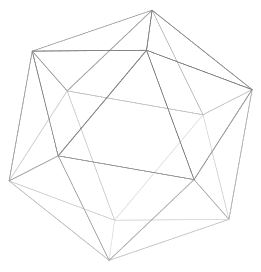Contents

# Contents

## Idea(image source)(image source)

The regular icosahedron is the unique regular convex polyhedron with 20 (triangular) faces. One of the Platonic solids.

Its symmetry group is called the icosahedral group.

$\,$

$\,$

$\,$

$\,$

$\,$

Dynkin diagram/
Dynkin quiver
dihedron,
Platonic solid
finite subgroups of SO(3)finite subgroups of SU(2)simple Lie group
$A_{n \geq 1}$cyclic group
$\mathbb{Z}_{n+1}$
cyclic group
$\mathbb{Z}_{n+1}$
special unitary group
$SU(n+1)$
A1cyclic group of order 2
$\mathbb{Z}_2$
cyclic group of order 2
$\mathbb{Z}_2$
SU(2)
A2cyclic group of order 3
$\mathbb{Z}_3$
cyclic group of order 3
$\mathbb{Z}_3$
SU(3)
A3
=
D3
cyclic group of order 4
$\mathbb{Z}_4$
cyclic group of order 4
$2 D_2 \simeq \mathbb{Z}_4$
SU(4)
$\simeq$
Spin(6)
D4dihedron on
bigon
Klein four-group
$D_4 \simeq \mathbb{Z}_2 \times \mathbb{Z}_2$
quaternion group
$2 D_4 \simeq$ Q8
SO(8), Spin(8)
D5dihedron on
triangle
dihedral group of order 6
$D_6$
binary dihedral group of order 12
$2 D_6$
SO(10), Spin(10)
D6dihedron on
square
dihedral group of order 8
$D_8$
binary dihedral group of order 16
$2 D_{8}$
SO(12), Spin(12)
$D_{n \geq 4}$dihedron,
hosohedron
dihedral group
$D_{2(n-2)}$
binary dihedral group
$2 D_{2(n-2)}$
special orthogonal group, spin group
$SO(2n)$, $Spin(2n)$
$E_6$tetrahedrontetrahedral group
$T$
binary tetrahedral group
$2T$
E6
$E_7$cube,
octahedron
octahedral group
$O$
binary octahedral group
$2O$
E7
$E_8$dodecahedron,
icosahedron
icosahedral group
$I$
binary icosahedral group
$2I$
E8
• Felix Klein, chater I.8 of Vorlesungen über das Ikosaeder und die Auflösung der Gleichungen vom fünften Grade, 1884, translated as Lectures on the Icosahedron and the Resolution of Equations of Degree Five by George Morrice 1888, online version

• Wikipedia, Icosahedron